# GSEB Solutions Class 11 Maths Chapter 15 Statistics Ex 15.2

Gujarat Board GSEB Textbook Solutions Class 11 Maths Chapter 15 Statistics Ex 15.2 Textbook Questions and Answers.

## Gujarat Board Textbook Solutions Class 11 Maths Chapter 15 Statistics Ex 15.2

Find the mean and variance for the following data in questions 1 to 5:
1. 6, 7, 10, 12, 13, 4, 8, 12
2. First n natural numbers.
3. First 10 multiples of 3.
4.5.Solutions to questions 1 to 5:
1. Mean $$\bar {x}$$ = $$\frac{\Sigma x_{i}}{n}$$ = $$\frac{6+7+10+12+13+4+8+12}{8}$$
= $$\frac{72}{8}$$ = 9.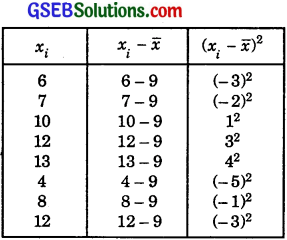∴ Σ(xi – $$\bar {x}$$ = 9 + 4 + 1 + 9 + 16 + 25 + 1 + 9 = 74.
∴ Variance = $$\frac{\Sigma\left(x_{i}-\bar{x}\right)^{2}}{\Sigma f_{i}}$$ = $$\frac{74}{8}$$ = 9.25.

2. The first n natural numbers are 1, 2, 3, …, n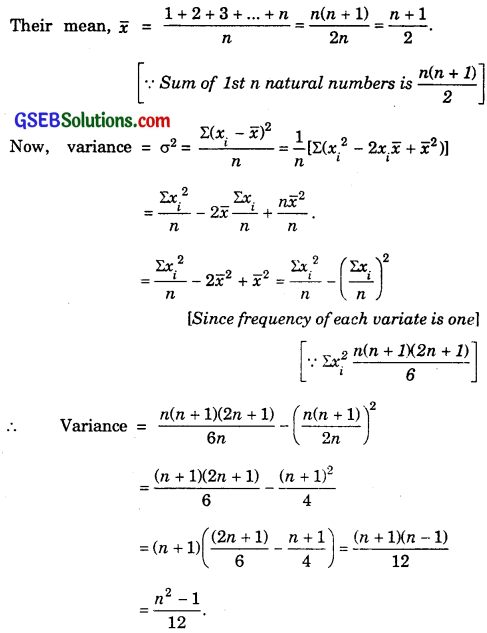3. First 10 multiples of 3 are 3, 6, 9, 12, 15, 18, 21, 24, 27, 30.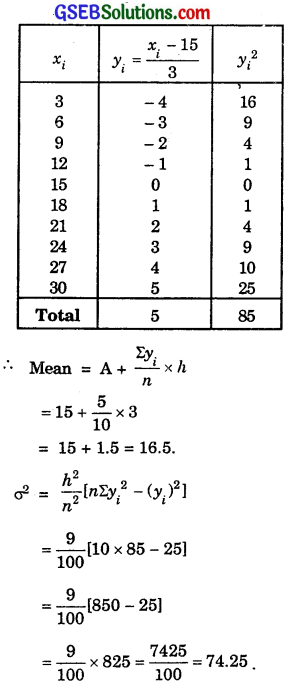Thus, mean = 16.5 and variance = 74.25.

4.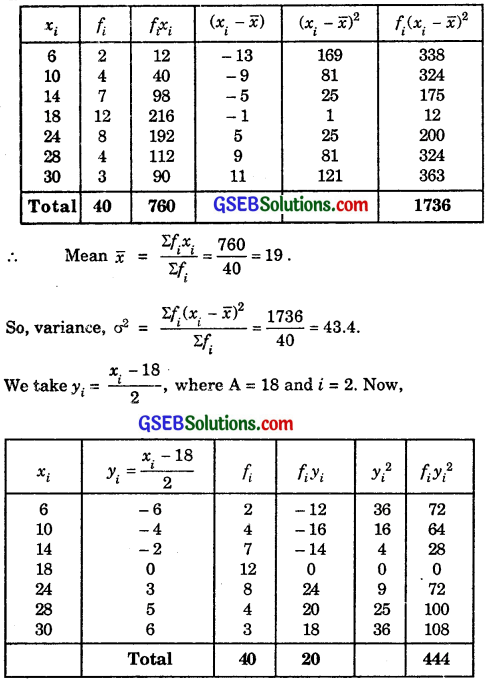Short-Cut Method:5.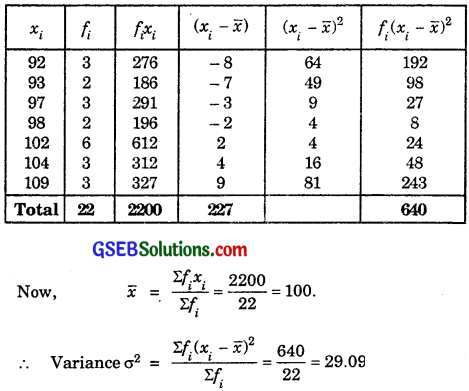Short-Cut Method:
Let yi = xi – 98, where we have assumed A = 98. Then,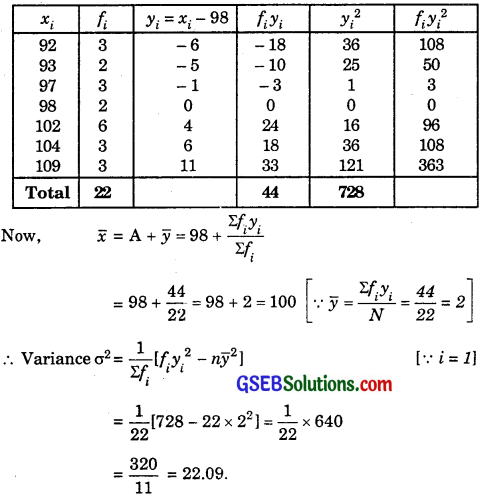Question 6.
Find the mean and standard deviation of the following, using short-cut method: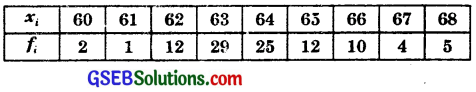Solution:∴ σ = $$\sqrt{2.86}$$ = 1.69.

Find the mean and variance for the following frequency distribution in questions 7 and 8:
7.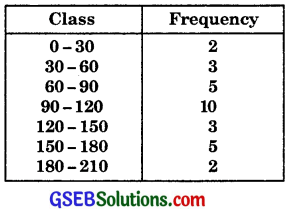8.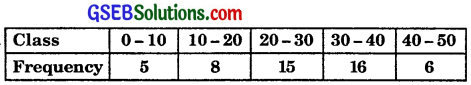Solutions to questions 7 and 8:= 30[76 – 0.13]
= 30 × 75.87 = 2276.

8.= 2(68 – 2) = 2 × 66 = 132.

Question 9.
Find the mean, variance and standard deviation of the following, using short-cut method:Solution: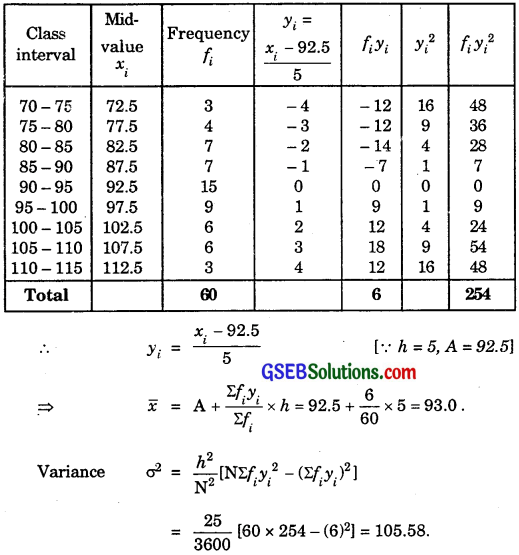∴ Standard deviation σ = $$\sqrt{105.58}$$ = 10.27.Question 10.
The diameter of circles (in mm) drawn in a design are given below: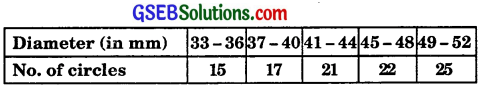Calculate the mean diameter and standard deviation of the circles.
Solution:
Firstly, the data is to be made continous by making classes as 32.5 – 36.5, 36.5 – 40.5, 40.5 – 44.5, 44.5 – 48.5, 48.5 – 52.5.= 5.55.